• Lectures
• Code
• Notebooks
• Community

# Asset Pricing I: Finite State Models¶

## Contents¶

“A little knowledge of geometric series goes a long way” – Robert E. Lucas, Jr.

“Asset pricing is all about covariances” – Lars Peter Hansen

## Overview¶

An asset is a claim on one or more future payoffs.

The spot price of an asset depends primarily on

• the anticipated dynamics for the stream of income accruing to the owners
• attitudes to risk
• rates of time preference

In this lecture we consider some standard pricing models and dividend stream specifications.

We study how prices and dividend-price ratios respond in these different scenarios.

We also look at creating and pricing derivative assets by repackaging income streams.

Key tools for the lecture are

• formulas for predicting future values of functions of a Markov state
• a formula for predicting the discounted sum of future values of a Markov state

## Pricing Models¶

In what follows let $\{d_t\}_{t \geq 0}$ be a stream of dividends.

• A time-$t$ cum-dividend asset is a claim to the stream $d_t, d_{t+1}, \ldots$.
• A time-$t$ ex-dividend asset is a claim to the stream $d_{t+1}, d_{t+2}, \ldots$.

Let’s look at some equations that we expect to hold for prices of assets under ex-dividend contracts (we will consider cum-dividend pricing in the exercises).

### Risk Neutral Pricing¶

Our first scenario is risk-neutral pricing.

Let $\beta = 1/(1+\rho)$ be an intertemporal discount factor, where $\rho$ is the rate at which agents discount the future.

The basic risk-neutral asset pricing equation for pricing one unit of an ex-dividend asset is.

$$p_t = \beta {\mathbb E}_t [d_{t+1} + p_{t+1}] \tag{1}$$

This is a simple “cost equals expected benefit” relationship.

Here ${\mathbb E}_t [y]$ denotes the best forecast of $y$, conditioned on information available at time $t$.

### Pricing with Random Discount Factor¶

What happens if for some reason traders discount payouts differently depending on the state of the world?

Michael Harrison and David Kreps [HK79] and Lars Peter Hansen and Scott Richard [HR87] showed that in quite general settings the price of an ex-dividend asset obeys

$$p_t = {\mathbb E}_t \left[ m_{t+1} ( d_{t+1} + p_{t+1} ) \right] \tag{2}$$

for some stochastic discount factor $m_{t+1}$.

The fixed discount factor $\beta$ in (1) has been replaced by the random variable $m_{t+1}$.

The way anticipated future payoffs are evaluated can now depend on various random outcomes.

One example of this idea is that assets that tend to have good payoffs in bad states of the world might be regarded as more valuable.

This is because they pay well when the funds are more urgently needed.

We give examples of how the stochastic discount factor has been modeled below.

### Asset Pricing and Covariances¶

Recall that, from the definition of a conditional covariance ${\rm cov}_t (x_{t+1}, y_{t+1})$, we have

$${\mathbb E}_t (x_{t+1} y_{t+1}) = {\rm cov}_t (x_{t+1}, y_{t+1}) + {\mathbb E}_t x_{t+1} {\mathbb E}_t y_{t+1} \tag{3}$$

If we apply this definition to the asset pricing equation (2) we obtain

$$p_t = {\mathbb E}_t m_{t+1} {\mathbb E}_t (d_{t+1} + p_{t+1}) + {\rm cov}_t (m_{t+1}, d_{t+1}+ p_{t+1}) \tag{4}$$

It is useful to regard equation (4) as a generalization of equation (1).

• In equation (1), the stochastic discount factor $m_{t+1} = \beta$, a constant.
• In equation (1), the covariance term ${\rm cov}_t (m_{t+1}, d_{t+1}+ p_{t+1})$ is zero because $m_{t+1} = \beta$.

Equation (4) asserts that the covariance of the stochastic discount factor with the one period payout $d_{t+1} + p_{t+1}$ is an important determinant of the price $p_t$.

We give examples of some models of stochastic discount factors that have been proposed later in this lecture and also in a later lecture.

### The Price-Dividend Ratio¶

Aside from prices, another quantity of interest is the price-dividend ratio $v_t := p_t / d_t$.

Let’s write down an expression that this ratio should satisfy.

We can divide both sides of (2) by $d_t$ to get

$$v_t = {\mathbb E}_t \left[ m_{t+1} \frac{d_{t+1}}{d_t} (1 + v_{t+1}) \right] \tag{5}$$

Below we’ll discuss the implication of this equation.

## Prices in the Risk Neutral Case¶

What can we say about price dynamics on the basis of the models described above?

The answer to this question depends on

1. the process we specify for dividends
2. the stochastic discount factor and how it correlates with dividends

For now let’s focus on the risk neutral case, where the stochastic discount factor is constant, and study how prices depend on the dividend process.

### Example 1: Constant dividends¶

The simplest case is risk neutral pricing in the face of a constant, non-random dividend stream $d_t = d > 0$.

Removing the expectation from (1) and iterating forward gives

\begin{aligned} p_t & = \beta (d + p_{t+1}) \\ & = \beta (d + \beta(d + p_{t+2})) \\ & \quad \vdots \\ & = \beta (d + \beta d + \beta^2 d + \cdots + \beta^{k-2} d + \beta^{k-1} p_{t+k}) \end{aligned}

Unless prices explode in the future, this sequence converges to

$$\bar p := \frac{\beta d}{1-\beta} \tag{6}$$

This price is the equilibrium price in the constant dividend case.

Indeed, simple algebra shows that setting $p_t = \bar p$ for all $t$ satisfies the equilibrium condition $p_t = \beta (d + p_{t+1})$.

### Example 2: Dividends with deterministic growth paths¶

Consider a growing, non-random dividend process $d_{t+1} = g d_t$ where $0 < g \beta < 1$.

While prices are not usually constant when dividends grow over time, the price dividend-ratio might be.

If we guess this, substituting $v_t = v$ into (5) as well as our other assumptions, we get $v = \beta g (1 + v)$.

Since $\beta g < 1$, we have a unique positive solution:

$$v = \frac{\beta g}{1 - \beta g }$$

The price is then

$$p_t = \frac{\beta g}{1 - \beta g } d_t$$

If, in this example, we take $g = 1+\kappa$ and let $\rho := 1/\beta - 1$, then the price becomes

$$p_t = \frac{1 + \kappa}{ \rho - \kappa} d_t$$

This is called the Gordon formula.

### Example 3: Markov growth, risk neutral pricing¶

Next we consider a dividend process

$$d_{t+1} = g_{t+1} d_t \tag{7}$$

The stochastic growth factor $\{g_t\}$ is given by

$$g_t = g(X_t), \quad t = 1, 2, \ldots$$

where

1. $\{X_t\}$ is a finite Markov chain with state space $S$ and transition probabilities
$$P(x, y) := \mathbb P \{ X_{t+1} = y \,|\, X_t = x \} \qquad (x, y \in S)$$
1. $g$ is a given function on $S$ taking positive values

You can think of

• $S$ as $n$ possible “states of the world” and $X_t$ as the current state
• $g$ as a function that maps a given state $X_t$ into a growth factor $g_t = g(X_t)$ for the endowment
• $\ln g_t = \ln (d_{t+1} / d_t)$ is the growth rate of dividends

(For a refresher on notation and theory for finite Markov chains see this lecture)

The next figure shows a simulation, where

• $\{X_t\}$ evolves as a discretized AR1 process produced using Tauchen’s method
• $g_t = \exp(X_t)$, so that $\ln g_t = X_t$ is the growth rate

### Setup¶

In :
using InstantiateFromURL
# optionally add arguments to force installation: instantiate = true, precompile = true
github_project("QuantEcon/quantecon-notebooks-julia", version = "0.8.0")

In :
using LinearAlgebra, Statistics
using Parameters, Plots, QuantEcon
gr(fmt = :png);

In :
n = 25
mc = tauchen(n, 0.96, 0.25)
sim_length = 80

x_series = simulate(mc, sim_length; init = round(Int, n / 2))
g_series = exp.(x_series)
d_series = cumprod(g_series) # assumes d_0 = 1

series = [x_series g_series d_series log.(d_series)]
labels = ["X_t" "g_t" "d_t" "ln(d_t)"]
plot(series, layout = 4, labels = labels)

Out: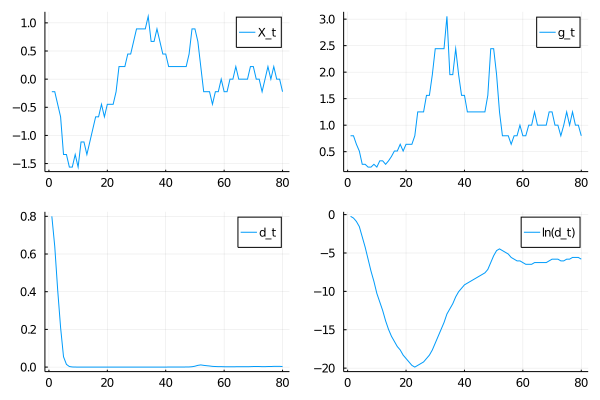#### Pricing¶

To obtain asset prices in this setting, let’s adapt our analysis from the case of deterministic growth.

In that case we found that $v$ is constant.

This encourages us to guess that, in the current case, $v_t$ is constant given the state $X_t$.

In other words, we are looking for a fixed function $v$ such that the price-dividend ratio satisfies $v_t = v(X_t)$.

We can substitute this guess into (5) to get

$$v(X_t) = \beta {\mathbb E}_t [ g(X_{t+1}) (1 + v(X_{t+1})) ]$$

If we condition on $X_t = x$, this becomes

$$v(x) = \beta \sum_{y \in S} g(y) (1 + v(y)) P(x, y)$$

or

$$v(x) = \beta \sum_{y \in S} K(x, y) (1 + v(y)) \quad \text{where} \quad K(x, y) := g(y) P(x, y) \tag{8}$$

Suppose that there are $n$ possible states $x_1, \ldots, x_n$.

We can then think of (8) as $n$ stacked equations, one for each state, and write it in matrix form as

$$v = \beta K (\mathbb 1 + v) \tag{9}$$

Here

• $v$ is understood to be the column vector $(v(x_1), \ldots, v(x_n))'$
• $K$ is the matrix $(K(x_i, x_j))_{1 \leq i, j \leq n}$
• ${\mathbb 1}$ is a column vector of ones

When does (9) have a unique solution?

From the Neumann series lemma and Gelfand’s formula, this will be the case if $\beta K$ has spectral radius strictly less than one.

In other words, we require that the eigenvalues of $K$ be strictly less than $\beta^{-1}$ in modulus.

The solution is then

$$v = (I - \beta K)^{-1} \beta K{\mathbb 1} \tag{10}$$

### Code¶

Let’s calculate and plot the price-dividend ratio at a set of parameters.

As before, we’ll generate $\{X_t\}$ as a discretized AR1 process and set $g_t = \exp(X_t)$.

Here’s the code, including a test of the spectral radius condition

In :
n = 25  # size of state space
β = 0.9
mc = tauchen(n, 0.96, 0.02)

K = mc.p .* exp.(mc.state_values)'

v = (I - β * K) \  (β * K * ones(n, 1))

plot(mc.state_values,
v,
lw = 2,
ylabel = "price-dividend ratio",
xlabel = "state",
alpha = 0.7,
label = "v")

Out: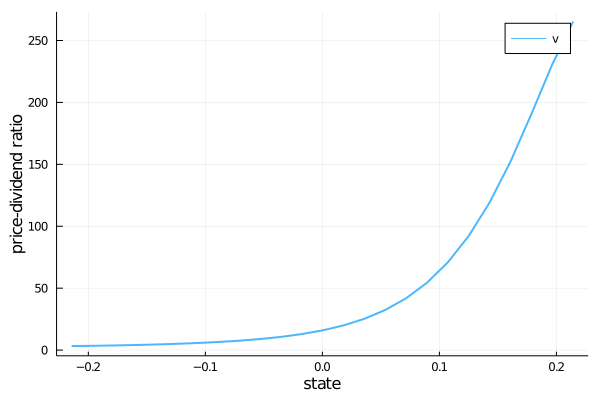Why does the price-dividend ratio increase with the state?

The reason is that this Markov process is positively correlated, so high current states suggest high future states.

Moreover, dividend growth is increasing in the state.

Anticipation of high future dividend growth leads to a high price-dividend ratio.

## Asset Prices under Risk Aversion¶

Now let’s turn to the case where agents are risk averse.

We’ll price several distinct assets, including

• The price of an endowment stream.
• A consol (a variety of bond issued by the UK government in the 19th century).
• Call options on a consol.

### Pricing a Lucas tree¶

Let’s start with a version of the celebrated asset pricing model of Robert E. Lucas, Jr. [Luc78].

As in [Luc78], suppose that the stochastic discount factor takes the form

$$m_{t+1} = \beta \frac{u'(c_{t+1})}{u'(c_t)} \tag{11}$$

where $u$ is a concave utility function and $c_t$ is time $t$ consumption of a representative consumer.

(A derivation of this expression is given in a later lecture)

Assume the existence of an endowment that follows (7).

The asset being priced is a claim on the endowment process.

Following [Luc78], suppose further that in equilibrium, consumption is equal to the endowment, so that $d_t = c_t$ for all $t$.

For utility, we’ll assume the constant relative risk aversion (CRRA) specification

$$u(c) = \frac{c^{1-\gamma}}{1 - \gamma} \ {\rm with} \ \gamma > 0 \tag{12}$$

When $\gamma =1$ we let $u(c) = \ln c$.

Inserting the CRRA specification into (11) and using $c_t = d_t$ gives

$$m_{t+1} = \beta \left(\frac{c_{t+1}}{c_t}\right)^{-\gamma} = \beta g_{t+1}^{-\gamma} \tag{13}$$

Substituting this into (5) gives the price-dividend ratio formula

$$v(X_t) = \beta {\mathbb E}_t \left[ g(X_{t+1})^{1-\gamma} (1 + v(X_{t+1}) ) \right]$$

Conditioning on $X_t = x$, we can write this as

$$v(x) = \beta \sum_{y \in S} g(y)^{1-\gamma} (1 + v(y) ) P(x, y)$$

If we let

$$J(x, y) := g(y)^{1-\gamma} P(x, y)$$

then we can rewrite in vector form as

$$v = \beta J ({\mathbb 1} + v )$$

Assuming that the spectral radius of $J$ is strictly less than $\beta^{-1}$, this equation has the unique solution

$$v = (I - \beta J)^{-1} \beta J {\mathbb 1} \tag{14}$$

We will define a function tree_price to solve for \$v\$ given parameters stored in the AssetPriceModel objects

In :
# A default Markov chain for the state process
ρ = 0.9
σ = 0.02
n = 25
default_mc = tauchen(n, ρ, σ)

AssetPriceModel = @with_kw (β = 0.96,
γ = 2.0,
mc = default_mc,
n = size(mc.p),
g = exp)

# test stability of matrix Q
function test_stability(ap, Q)
sr = maximum(abs, eigvals(Q))
if sr ≥ 1 / ap.β
msg = "Spectral radius condition failed with radius = $sr" throw(ArgumentError(msg)) end end # price/dividend ratio of the Lucas tree function tree_price(ap; γ = ap.γ) # Simplify names, set up matrices @unpack β, mc = ap P, y = mc.p, mc.state_values y = reshape(y, 1, ap.n) J = P .* ap.g.(y).^(1 - γ) # Make sure that a unique solution exists test_stability(ap, J) # Compute v v = (I - β * J) \ sum(β * J, dims = 2) return v end  Out: tree_price (generic function with 1 method) Here’s a plot of$ v $as a function of the state for several values of$ \gamma $, with a positively correlated Markov process and$ g(x) = \exp(x) $In : γs = [1.2, 1.4, 1.6, 1.8, 2.0] ap = AssetPriceModel() states = ap.mc.state_values lines = [] labels = [] for γ in γs v = tree_price(ap, γ = γ) label = "gamma =$γ"
push!(labels, label)
push!(lines, v)
end

plot(lines,
labels = reshape(labels, 1, length(labels)),
title = "Price-dividend ratio as a function of the state",
ylabel = "price-dividend ratio",
xlabel = "state")

Out: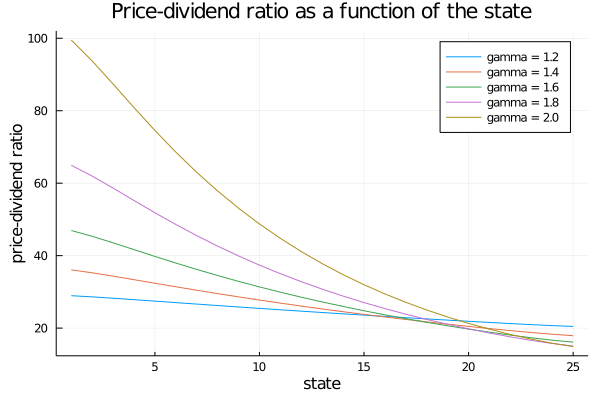Notice that $v$ is decreasing in each case.

This is because, with a positively correlated state process, higher states suggest higher future consumption growth.

In the stochastic discount factor (13), higher growth decreases the discount factor, lowering the weight placed on future returns.

#### Special cases¶

In the special case $\gamma =1$, we have $J = P$.

Recalling that $P^i {\mathbb 1} = {\mathbb 1}$ for all $i$ and applying Neumann’s geometric series lemma, we are led to

$$v = \beta(I-\beta P)^{-1} {\mathbb 1} = \beta \sum_{i=0}^{\infty} \beta^i P^i {\mathbb 1} = \beta \frac{1}{1 - \beta} {\mathbb 1}$$

Thus, with log preferences, the price-dividend ratio for a Lucas tree is constant.

Alternatively, if $\gamma = 0$, then $J = K$ and we recover the risk neutral solution (10).

This is as expected, since $\gamma = 0$ implies $u(c) = c$ (and hence agents are risk neutral).

### A Risk-Free Consol¶

Consider the same pure exchange representative agent economy.

A risk-free consol promises to pay a constant amount $\zeta> 0$ each period.

Recycling notation, let $p_t$ now be the price of an ex-coupon claim to the consol.

An ex-coupon claim to the consol entitles the owner at the end of period $t$ to

• $\zeta$ in period $t+1$, plus
• the right to sell the claim for $p_{t+1}$ next period

The price satisfies (2) with $d_t = \zeta$, or

$$p_t = {\mathbb E}_t \left[ m_{t+1} ( \zeta + p_{t+1} ) \right]$$

We maintain the stochastic discount factor (13), so this becomes

$$p_t = {\mathbb E}_t \left[ \beta g_{t+1}^{-\gamma} ( \zeta + p_{t+1} ) \right] \tag{15}$$

Guessing a solution of the form $p_t = p(X_t)$ and conditioning on $X_t = x$, we get

$$p(x) = \beta \sum_{y \in S} g(y)^{-\gamma} (\zeta + p(y)) P(x, y)$$

Letting $M(x, y) = P(x, y) g(y)^{-\gamma}$ and rewriting in vector notation yields the solution

$$p = (I - \beta M)^{-1} \beta M \zeta {\mathbb 1} \tag{16}$$

The above is implemented in the function consol_price

In :
function consol_price(ap, ζ)
# Simplify names, set up matrices
@unpack β, γ, mc, g, n = ap
P, y = mc.p, mc.state_values
y = reshape(y, 1, n)
M = P .* g.(y).^(-γ)

# Make sure that a unique solution exists
test_stability(ap, M)

# Compute price
return (I - β * M) \ sum(β * ζ * M, dims = 2)
end

Out:
consol_price (generic function with 1 method)

### Pricing an Option to Purchase the Consol¶

Let’s now price options of varying maturity that give the right to purchase a consol at a price $p_S$.

#### An infinite horizon call option¶

We want to price an infinite horizon option to purchase a consol at a price $p_S$.

The option entitles the owner at the beginning of a period either to

1. purchase the bond at price $p_S$ now, or
2. Not to exercise the option now but to retain the right to exercise it later

Thus, the owner either exercises the option now, or chooses not to exercise and wait until next period.

This is termed an infinite-horizon call option with strike price $p_S$.

The owner of the option is entitled to purchase the consol at the price $p_S$ at the beginning of any period, after the coupon has been paid to the previous owner of the bond.

The fundamentals of the economy are identical with the one above, including the stochastic discount factor and the process for consumption.

Let $w(X_t, p_S)$ be the value of the option when the time $t$ growth state is known to be $X_t$ but before the owner has decided whether or not to exercise the option at time $t$ (i.e., today).

Recalling that $p(X_t)$ is the value of the consol when the initial growth state is $X_t$, the value of the option satisfies

$$w(X_t, p_S) = \max \left\{ \beta \, {\mathbb E}_t \frac{u'(c_{t+1})}{u'(c_t)} w(X_{t+1}, p_S), \; p(X_t) - p_S \right\}$$

The first term on the right is the value of waiting, while the second is the value of exercising now.

We can also write this as

$$w(x, p_S) = \max \left\{ \beta \sum_{y \in S} P(x, y) g(y)^{-\gamma} w (y, p_S), \; p(x) - p_S \right\} \tag{17}$$

With $M(x, y) = P(x, y) g(y)^{-\gamma}$ and $w$ as the vector of values $(w(x_i), p_S)_{i = 1}^n$, we can express (17) as the nonlinear vector equation

$$w = \max \{ \beta M w, \; p - p_S {\mathbb 1} \} \tag{18}$$

To solve (18), form the operator $T$ mapping vector $w$ into vector $Tw$ via

$$T w = \max \{ \beta M w,\; p - p_S {\mathbb 1} \}$$

Start at some initial $w$ and iterate to convergence with $T$.

We can find the solution with the following function call_option

In :
# price of perpetual call on consol bond
function call_option(ap, ζ, p_s, ϵ = 1e-7)

# Simplify names, set up matrices
@unpack β, γ, mc, g, n = ap
P, y = mc.p, mc.state_values
y = reshape(y, 1, n)
M = P .* g.(y).^(-γ)

# Make sure that a unique console price exists
test_stability(ap, M)

# Compute option price
p = consol_price(ap, ζ)
w = zeros(ap.n, 1)
error = ϵ + 1
while (error > ϵ)
# Maximize across columns
w_new = max.(β * M * w, p .- p_s)
# Find maximal difference of each component and update
error = maximum(abs, w - w_new)
w = w_new
end

return w
end

Out:
call_option (generic function with 2 methods)

Here’s a plot of $w$ compared to the consol price when $P_S = 40$

In :
ap = AssetPriceModel(β=0.9)
ζ = 1.0
strike_price = 40.0

x = ap.mc.state_values
p = consol_price(ap, ζ)
w = call_option(ap, ζ, strike_price)

plot(x, p, color = "blue", lw = 2, xlabel = "state", label = "consol price")
plot!(x, w, color = "green", lw = 2, label = "value of call option")

Out: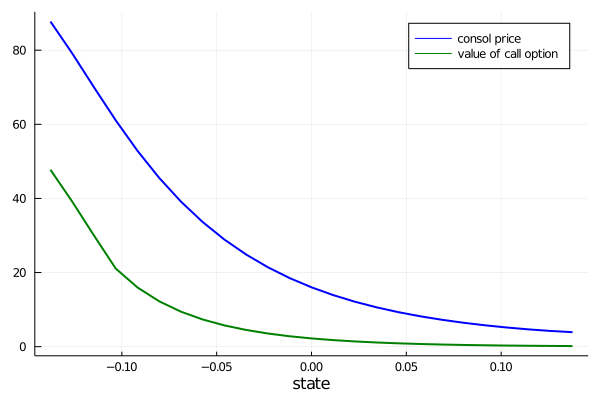In large states the value of the option is close to zero.

This is despite the fact the Markov chain is irreducible and low states — where the consol prices is high — will eventually be visited.

The reason is that $\beta=0.9$, so the future is discounted relatively rapidly

### Risk Free Rates¶

Let’s look at risk free interest rates over different periods.

#### The one-period risk-free interest rate¶

As before, the stochastic discount factor is $m_{t+1} = \beta g_{t+1}^{-\gamma}$.

It follows that the reciprocal $R_t^{-1}$ of the gross risk-free interest rate $R_t$ in state $x$ is

$${\mathbb E}_t m_{t+1} = \beta \sum_{y \in S} P(x, y) g(y)^{-\gamma}$$

We can write this as

$$m_1 = \beta M {\mathbb 1}$$

where the $i$-th element of $m_1$ is the reciprocal of the one-period gross risk-free interest rate in state $x_i$.

#### Other terms¶

Let $m_j$ be an $n \times 1$ vector whose $i$ th component is the reciprocal of the $j$ -period gross risk-free interest rate in state $x_i$.

Then $m_1 = \beta M$, and $m_{j+1} = M m_j$ for $j \geq 1$.

## Exercises¶

### Exercise 1¶

In the lecture, we considered ex-dividend assets.

A cum-dividend asset is a claim to the stream $d_t, d_{t+1}, \ldots$.

Following (1), find the risk-neutral asset pricing equation for one unit of a cum-dividend asset.

With a constant, non-random dividend stream $d_t = d > 0$, what is the equilibrium price of a cum-dividend asset?

With a growing, non-random dividend process $d_t = g d_t$ where $0 < g \beta < 1$, what is the equilibrium price of a cum-dividend asset?

### Exercise 2¶

Consider the following primitives

In :
n = 5
P = fill(0.0125, n, n) + (0.95 - 0.0125)I
s = [1.05, 1.025, 1.0, 0.975, 0.95]
γ = 2.0
β = 0.94
ζ = 1.0

Out:
1.0

Let $g$ be defined by $g(x) = x$ (that is, $g$ is the identity map).

Compute the price of the Lucas tree.

Do the same for

• the price of the risk-free consol when $\zeta = 1$
• the call option on the consol when $\zeta = 1$ and $p_S = 150.0$

### Exercise 3¶

Let’s consider finite horizon call options, which are more common than the infinite horizon variety.

Finite horizon options obey functional equations closely related to (17).

A $k$ period option expires after $k$ periods.

If we view today as date zero, a $k$ period option gives the owner the right to exercise the option to purchase the risk-free consol at the strike price $p_S$ at dates $0, 1, \ldots , k-1$.

The option expires at time $k$.

Thus, for $k=1, 2, \ldots$, let $w(x, k)$ be the value of a $k$-period option.

It obeys

$$w(x, k) = \max \left\{ \beta \sum_{y \in S} P(x, y) g(y)^{-\gamma} w (y, k-1), \; p(x) - p_S \right\}$$

where $w(x, 0) = 0$ for all $x$.

We can express the preceding as the sequence of nonlinear vector equations

$$w_k = \max \{ \beta M w_{k-1}, \; p - p_S {\mathbb 1} \} \quad k =1, 2, \ldots \quad \text{with } w_0 = 0$$

Write a function that computes $w_k$ for any given $k$.

Compute the value of the option with k = 5 and k = 25 using parameter values as in Exercise 1.

Is one higher than the other? Can you give intuition?

## Solutions¶

### Exercise 2¶

In :
n = 5
P = fill(0.0125, n, n) + (0.95 - 0.0125)I
s = [0.95, 0.975, 1.0, 1.025, 1.05]  # state values
mc = MarkovChain(P, s)

γ = 2.0
β = 0.94
ζ = 1.0
p_s = 150.0

Out:
150.0

Next we’ll create an instance of AssetPriceModel to feed into the functions.

In :
ap = AssetPriceModel(β = β, mc = mc, γ = γ, g = x -> x)

Out:
(β = 0.94, γ = 2.0, mc = Discrete Markov Chain
stochastic matrix of type Array{Float64,2}:
[0.95 0.0125 … 0.0125 0.0125; 0.0125 0.95 … 0.0125 0.0125; … ; 0.0125 0.0125 … 0.95 0.0125; 0.0125 0.0125 … 0.0125 0.95], n = 5, g = var"#5#6"())
In :
v = tree_price(ap)
println("Lucas Tree Prices: $v\n")  Lucas Tree Prices: [29.474015777145; 21.935706611219704; 17.571422357262904; 14.725150017725884; 12.722217630644252]  In : v_consol = consol_price(ap, 1.0) println("Consol Bond Prices:$(v_consol)\n")

Consol Bond Prices: [753.8710047641987; 242.55144081989457; 148.67554548466478; 109.25108965024712; 87.56860138531121]


In :
w = call_option(ap, ζ, p_s)

Out:
5×1 Array{Float64,2}:
603.8710047641987
176.839334301913
108.67734499337003
80.05179254233045
64.30843748377002

### Exercise 3¶

Here’s a suitable function:

In :
function finite_horizon_call_option(ap, ζ, p_s, k)

# Simplify names, set up matrices
@unpack β, γ, mc = ap
P, y = mc.p, mc.state_values
y = y'
M = P .* ap.g.(y).^(- γ)

# Make sure that a unique console price exists
test_stability(ap, M)

# Compute option price
p = consol_price(ap, ζ)
w = zeros(ap.n, 1)
for i in 1:k
# Maximize across columns
w = max.(β * M * w, p .- p_s)
end

return w
end

Out:
finite_horizon_call_option (generic function with 1 method)
In :
lines = []
labels = []
for k in [5, 25]
w = finite_horizon_call_option(ap, ζ, p_s, k)
push!(lines, w)
push!(labels, "k = $k") end plot(lines, labels = reshape(labels, 1, length(labels)))  Out: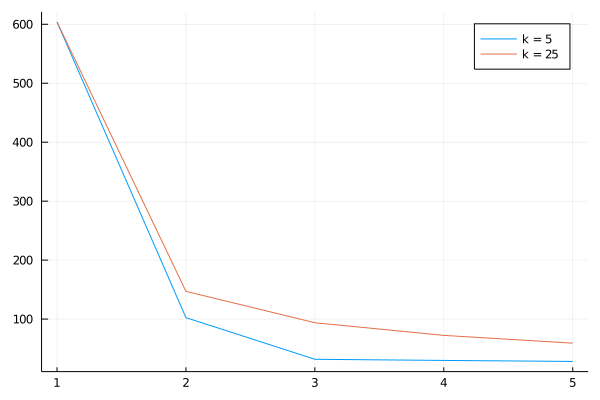Not surprisingly, the option has greater value with larger$ k \$. This is because the owner has a longer time horizon over which he or she may exercise the option.

• Share page